Triangle Congruence Part 1
Triangle Congruence Part 2
Triangle Congruence Part 3
Triangle Congruence Part 4
Ms. Buxton
100

The two triangles are congruent by the following...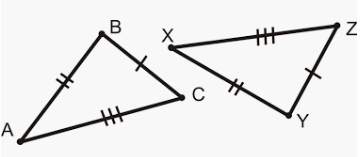SSS

100

The following triangles are congruent by...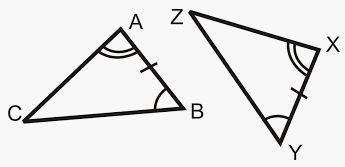ASA

100

A triangle that has all three sides congruent.

Equilateral Triangle

100

3 acute angles

acute triangle

100

What town is Ms. Buxton from

Fair Haven

200

The following triangles are congruent by...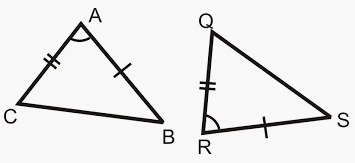SAS

200

The following triangles are congruent by...AAS

200

splits an isosceles triangle into 2 right triangles

perpendicular bisector

200

obtuse triangle

1 obtuse angle

200

What month is Ms. Buxton's birthday

November

300

Are the following triangles congruent?  If yes, by which theorem?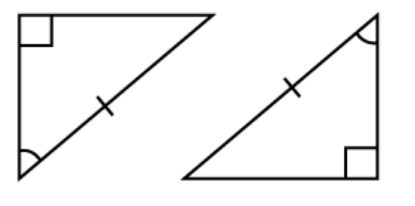AAS

300

What else additional information is needed to prove triangle PQS  is congruent to triangle RQS   using SAS?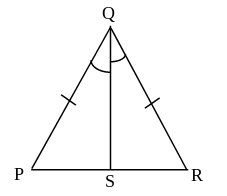Side QS is congruent to Side QS

300

splits a segment into 2 congruent parts

midpoint

300

1 right angle

right triangle

300

What bone did Ms. Buxton break in kindergarten

Her forearm (arm)

400

What additional information would be needed in order to prove the two triangles are congruent using HL theorem?Angle F = 90 degrees.

400

What additional information is needed to prove the triangles UST and USV are congruent using HL theorem? Be specific.US = US or US is a perpendicular bisector of TV

UT = UT

400

A triangle with two congruent sides.

Isosceles Triangle

400

cpctc

corresponding parts of congruent triangles are congruent

400

Where was Ms. Buxton born

North Carolina
500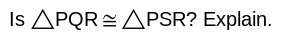Yes because PR = PR therefore the triangles can be proven through SAS

500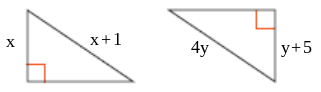x = 7, y = 2

500

A triangle that has no congruent sides.

Scalene Triangle

500

isosceles triangle

exactly 2 congruent sides

500

What does Ms. Buxton drive?

Subaru Impreza

Click to zoom